2015-04-16 11:14:41 c602273091 阅读数 9252
• ###### 自然语言处理——实战分词

1245 人正在学习 去看看 魏俊

# 基础

【1】了解HMM算法：http://www.cnblogs.com/pangxiaodong/archive/2011/10/17/2214542.html

【2】再次加深印象：http://blog.csdn.net/likelet/article/details/7056068

# 代码：

免积分下载：

【6】http://blog.csdn.net/luxiaoxun/article/details/7726423    这是几种当前比较流行的开源代码

2020-02-06 19:44:45 weixin_38719187 阅读数 259
• ###### 自然语言处理——实战分词

1245 人正在学习 去看看 魏俊1、读取语音
global filep filename
[filename,filepath]=uigetfile(’*.wav’,‘选择音频文件’);
filep=strcat(filepath,filename);
namen3=filename;
D = dir(filep);
set(handles.edit1,‘string’,num2str(namen3));
namen4=[‘文件路径：’,num2str(filep),10, ‘文件名：’,num2str(D.name),10,‘采样频率：’,num2str(fs1),10,‘文件大小：’,num2str(D.bytes),‘bytes’,10];
set(handles.listbox1,‘string’,namen4);
axes(handles.axes1);
plot(y1);
namen=‘原始语音信号’;
set(handles.text2,‘string’,num2str(namen));

2、语音的播放
global filep
sound(filex,fs);

3、识别
global filep hmm
O = mfcc(x);
O = O(x1:x2-5,:);

``````for j=1:10
pout(j) = viterbi(hmm{j}, O);%调用自定义的子函数viterbi.m
end
[d,n] = max(pout);
``````

% delete(d);
c=num2str(n-1);
set(handles.edit5,‘string’,c);
guidata(hObject, handles);
4、识别率的计算
a=zeros(1,30);b=[zeros(1,3),ones(1,3),2ones(1,3),3ones(1,3),4ones(1,3),5ones(1,3),6ones(1,3),7ones(1,3),8ones(1,3),9ones(1,3)];
for i=1:30
fname = sprintf(‘test1\%d.wav’,i);
M=handles.M;
y = awgn(k,M,‘measured’);%此为加噪声函数，第二个参数是噪声值。
O = mfcc(y);
O = O(x1:x2-5,:);
for j=1:10
pout(j) = viterbi(hmm{j}, O);

`````` end
[d,n] = max(pout);
a(1,i)=n-1;
``````

end
z=a-b;
d=sum(z==0);
e=d/30;
% fprintf(‘识别率为%d\n’, e);
set(handles.edit4,‘string’,num2str(e));
guidata(hObject,handles);

2017-09-08 22:00:11 qq_37572875 阅读数 16587
• ###### 自然语言处理——实战分词

1245 人正在学习 去看看 魏俊

0 语音识别概述 1
1 语音识别的算法 2
1.1.1 DNN-HMM 2
1.1.2 RNN-CTC 3
1.1.4FSMN 3
1.1.5 LSTM-DNN电话交谈语音识别 3
1.1.6Android科大讯飞语音识别源码及API下载 3
2. 语音识别的应用场景 4
2.1语音识别的智能家居框架 4
2.2移动端的使用 5
2.3内容监管上的运用 6
2.3.1 语音识别技术应用之音频切分和分类技术 6
2.3.2 语音识别技术应用之音频模板匹配技术 6
2.3.3 语音识别技术应用之节目的自动发现技术 7
2.4 语音导航系统 7
2.4.1 语音导航系统构架 7
2.5 医疗领域 9
2.6 在社交方面的运用 9
3 语音识别数据集 11
4 语音识别行业分析 12
4.1 智能语音技术取得重大突破，商业化落地成为可能 12
4.2 智能车载、智能家居及可穿戴设备风潮的兴起加速语音技术落地 13
4.3科技巨头，初创公司纷纷从不同维度布局相关产业链 14
4.4 面向物联网的智能语音产业链的形成将引起商业模式的变化 16

# 0 语音识别概述

http://pan.baidu.com/s/1jHI22DS

https://www.leiphone.com/news/201608/4HJoePG2oQfGpoj2.html

http://pan.baidu.com/s/1mhFG7xu
CNN在语音识别上的应用：
http://www.52cs.org/?p=1870

https://www.jiqizhixin.com/articles/2016-11-04-4

## 1 语音识别的算法

1.1.1 DNN-HMMDNN-HMM模型

http://blog.csdn.net/xmdxcsj/article/details/52760080

https://github.com/wenjiegroup/DNN-HMM/tree/master/Codes_packaging

1.1.4FSMN
Python代码：
https://github.com/katsugeneration/tensor-fsmn/blob/master/ptb.py

1.1.5 LSTM-DNN电话交谈语音识别
http://pan.baidu.com/s/1eRC4ZdC
LSTM介绍以及程序实现：https://zybuluo.com/hanbingtao/note/581764

# 2.语音识别的应用场景

## 2.1语音识别的智能家居框架CMU Sphinx 提供的类库. 主要功能包含语音识别算法和语义转换模块, 它被部署在 Java 虚拟机上. 语音识别算法的主要过程有: 语音输入、预处理、特征提取、模型匹配、输出结果. 首先必须使用 CMU Sphinx的训练工具以特定声学模型为基础对语料库获取匹配的 MFCC 特征数据, 然后使用 MAP 和 MLLR 自适应技术来改进原来的声学模型。

## 2.3内容监管上的运用

2.3.1 语音识别技术应用之音频切分和分类技术

2.3.2 语音识别技术应用之音频模板匹配技术

2.3.3 语音识别技术应用之节目的自动发现技术

## 2.4 语音导航系统

2.4.1 语音导航系统构架1）客户通过手机或者固话，利用排队机接入呼叫中心系统，在 CTI 和 IVR 的控制下，当用户需要语音导航业务时，通过呼叫平台实现话务接入，平台记录下的原始语音文本，并进行播报用户确认无误后，将该文件作为语音识别引擎的输入；
2）原始的语音文件信息经过语音识别模块，分别经过特征提取、端点检测、去燥处理等语音识别预处理技术，经过处理过的较为干净的语音文件在经过离线解码、在线解码、置信度等处理，转出成自然语言文本信息，并将原始文件信息、原始语音信息、语音特征信息存入文本 / 语音语库中。
3）将自然语言文本信息经过自然语言处理，分别经过模式匹配、语法分析、语义解析、语义搜索、上下文管理、预测等技术处理，将自然语言文本信息转换成计算机能识别的语音信息作为输出物。
4）并进行业务需求的分析，为自然语言处理引擎提供关键数据输入。

## 2.6 在社交方面的运用

A、熟人通讯方面，我们应该都见过这样的场景，出租车上司机们通过对讲平台互相勾兑、插科打诨。路上匆忙的低头族，很多都在回微信，而如果通过声控交互构建一个对讲平台，这个体验就完全不一样了，你不需要用眼和手也可以随时给某某捎去一段话，别人给你发来语音、声控交互会提示你是否接听，而如果这个交互可以通过耳麦自动识别微小的语音（并根据接听者情况适度放大），那么以后走在路上就可以轻松而不违和地与人通话了，这个事情的意义在于将从另一个角度接管用户的关系链，形成大网优势。
B、陌生人社交方面，当下社交产品最大的痛点就是社交质量差、低效社交多、效率远远赶不上用户预期，一个突出表现就是搭讪无人理睬、理睬也在若干小时之后（所以人们为何喜欢直播，因为美女主播至少会说谢谢反馈下），但是语音沟通是一个更加强调即时性和真实性的场景，又不像视频那么需要“化妆和端着”，所以反而可能成为新的突破口。至于当下的语音社交平台为何多数做的不好，因为太像色情声讯台了！
C、兴趣推荐。如果选择值得一听的声音节目成本如此之高，那么一个比较好的办法就是基于兴趣的推荐来降低选择难度。比如喜马拉雅已经推出了“猜你喜欢”系统，可以通过深度学习、进行类似今日头条那样的个性化推荐。那么再进一步，如果以后这个推荐可以不需要通过眼睛完成呢，直接传来一个声音：“小喜根据你的爱好搜到了几个节目，下面念下标题，你感兴趣就喊要这个……”
D、时空触发。这个意思是，应该给更多的信息赋予时空属性，比如在某一时刻放出，或者在某一位置放出。举一个例子，你今天刚到一家商场，连上wifi，耳麦里立刻提醒你有最新的优惠活动信息、要不要听。你像即刻那样设置一些关注节点，比如某股又跌了，当这个消息到来，耳麦里立刻提醒你要不要听。你到达某个景点，耳边响起景点的典故。你晚上睡不着了，耳边响起周围人的声音，像不像《her》？
E、做强参与。同为视频，直播爆火的劲头远远强于当年的视频平台和短视频，这也释放了一个信号，用户并不满足于成为单向的内容接受者，他们也希望成为内容的参与者甚至再创作者，他们也希望得到更多的互动和回馈，来满足参与感、存在感和归属感，所以类似电台的互动直播很重要。

# 4 语音识别行业分析

## 4.1 智能语音技术取得重大突破，商业化落地成为可能

◈智能语音技术是人工智能产业链上的关键一环

◈ 中国人工智能市场规模持续增长，智能语音将居于重要地位
◈ 智能语音技术成熟，商业化应用成为可能

◈ 商业场景落地的重要环节语音交互有了重大突破

## 4.2 智能车载、智能家居及可穿戴设备风潮的兴起加速语音技术落地

◈ 智能语音市场整体处于启动期，智能车载，智能家居，智能可穿戴等垂直领域处于爆发前夜
◈ 智能车载市场前景广阔，预计五年内车联网渗透率超过50%，语音将成为车载系统标配
◈ 智能家电渗透率提高，智能家居市场蕴涵千亿市场规模，语音作为家居交互入口将大有所为

## 4.3科技巨头，初创公司纷纷从不同维度布局相关产业链

◈ 国外科技巨头：通过并购等手段，夯实核心技术，开放应用平台，在既有的产品和业务中实现AI first，扩展以AI为核心的生态系统

◈ 苹果：基于智能硬件定标准、做平台、获数据 ，重视物联网时代生态控制权

◈ 国内互联网巨头：开放语音生态系统，以产业内合作的方式，将语音技术植入产品和或应用于相关业务场景，构建全产业生态链

◈ 百度：瞄准人工智能战场，对外开放语音生态系统，对内在自身产品业务中实现AI First
◈ 国内智能语音公司：依托原有优势，从单一智能语音技术商转型全方位人工智能技术服务商
◈ 科大讯飞：传统优势明显，未来将更注重通用人工智能技术和平台级业务的拓展

◈ 初创厂商：以垂直领域和细分场景为突破口，重点布局家居，车载和可穿戴设备
◈ 图灵机器人：定位于语义和认知计算的平台服务提供商，提供聊天机器人平台和机器人操作系统

## 4.4 面向物联网的智能语音产业链的形成将引起商业模式的变化

◈ 未来趋势：以语音为入口，建立以物联网为基础的商业模式
◈ 智能家居：以合适的入口级应用为载体，基于万物互联的标准，将技术与硬件结合，实现内容和服务的拓展
◈ 智能车载：车联网向纵深方向发展，硬件基础功能免费，基于用户数据的挖掘和增值服务将成为未来主要赢利点

《新型工业化》开放式获取期刊：www.chinaxxgyh.com

2018-06-13 20:04:10 u010866505 阅读数 6751
• ###### 自然语言处理——实战分词

1245 人正在学习 去看看 魏俊

GMM-HMM语音识别原理

1.       HMM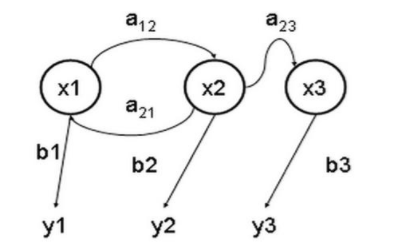a：转移概率

b：输出概率

y：观测状态

x：隐含状态

A:   转移概率

B:   输出概率

O：观测状态

S：隐含状态

1.       已知隐含状态数目，已知转移概率，根据观测状态，估计观测状态对应的隐含状态

2.       已知隐含状态数目，已知转移概率，根据观测状态，估计输出这个观测状态的概率

3.       已知隐含状态数目，根据大量实验结果(很多组观测状态)，反推转移概率

1)       在语音处理中，一个word有一个或多个音素构成。怎么说呢？这里补充一下语言学的一些知识。在语言学中,word(字)还可以再分解成音节(syllable)，音节可以再分成音素(phoneme)，音素之后就不能再分了.因此音素是语音中最小的单元，不管语音识别还是语音合成,都是在最小单元上进行操作的,即phoneme。比如我们的“我”，它的拼音是wo3(这个其实就是word，即字)，由于中文的word和syllable是相同的，即中文是单音节语言，即中文有且只有一个音节，但英文就不一样,比如hello这个单词，他就是有两个音节,hello=hae|low,即hello有hae和low这两个音节组成.音节下一层是phoneme(音素),语音识别的过程就是把这些 音素找到，然后音素拼接成音节，音节在拼接成word.如下图所示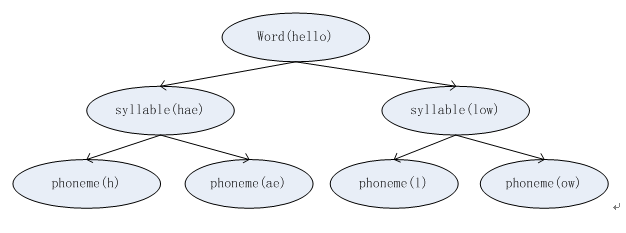2)       在训练时，一个HMM对应一个音素,每个HMM包含n个state(状态)，有的是3个状态,有的是5个状态，状态数在训练前确定后，一旦训练完成所有HMM的状态个数都是一致的，比如3个。

GMM是当做发射概率的，即在已知观测状态情况下,哪一种音素会产生这种状态的概率,概率最大的就是我们要的音素。因此，GMM是来计算某一个音素的概率。

GMM的全称是gaussmixture model(高斯混合模型),在训练前，一般会定义由几个高斯来决定音素的概率(高斯数目是超参数)。如下图所示为3高斯：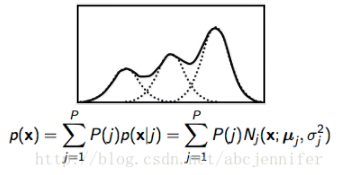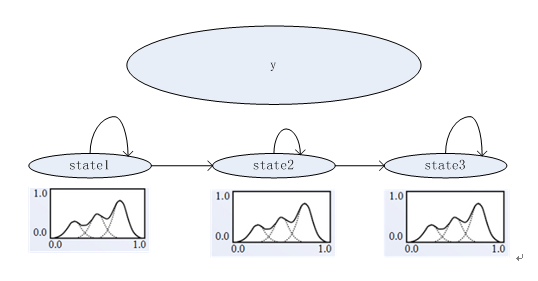1:将送进来的语音信号进行分帧(一般是20ms一帧，帧移是10ms)，然后提取特征

2：用GMM，判断当前的特征序列属于哪个状态(计算概率)

3：根据前面两个步骤，得出状态序列，其实就得到了音素序列，即得到了声韵母序列。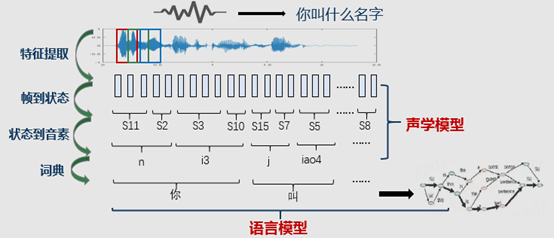1:gParam.py,主要是为了配置一些参数

2:核心文件是my_hmm.py，里面实现的是主要代码。

3:test.py是运行文件.

wav数据的格式是：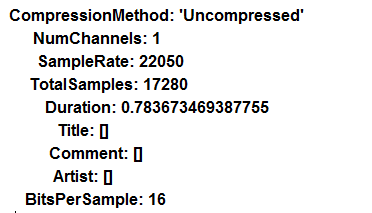gParam.py 代码解析:

```#! /usr/bin python
# encoding:utf-8

TRAIN_DATA_PATH = './data/train/'
TEST_DATA_PATH = './data/test/'
NSTATE = 4
NPDF = 3
MAX_ITER_CNT = 100
NUM=10```

```#! /usr/bin python
# encoding:utf_8

import numpy as np
from numpy import *
from sklearn.cluster import KMeans
from scipy import sparse
import scipy.io as sio
from scipy import signal
import wave
import math
import gParam
import copy

def pdf(m,v,x):
'''计算多元高斯密度函数
输入:
m---均值向量 SIZE×1
v---方差向量 SIZE×1
x---输入向量 SIZE×1
输出:
p---输出概率'''
test_v = np.prod(v,axis=0)
test_x = np.dot((x-m)/v,x-m)
p = (2*math.pi*np.prod(v,axis=0))**-0.5*np.exp(-0.5*np.dot((x-m)/v,x-m))
return p

# class of every sample infomation
class sampleInfo:
"""docstring for ClassName"""
def __init__(self):
self.smpl_wav = []
self.smpl_data = []
self.seg = []
def set_smpl_wav(self,wav):
self.smpl_wav.append(wav)
def set_smpl_data(self,data):
self.smpl_data.append(data)
def set_segment(self, seg_list):
self.seg = seg_list

#class of mix info from KMeans
class mixInfo:
"""docstring for mixInfo"""
def __init__(self):
self.Cmean = []
self.Cvar = []
self.Cweight = []
self.CM = []
class hmmInfo:
'''hmm model param'''
def __init__(self):
self.init = [] #初始矩阵
self.trans = [] #转移概率矩阵
self.mix = [] #高斯混合模型参数
self.N = 0 #状态数
# class of gmm_hmm model
class gmm_hmm:
def __init__(self):
self.hmm = [] #单个hmm序列,
self.gmm_hmm_model = [] #把所有的训练好的gmm-hmm写入到这个队列
self.samples = [] # 0-9 所有的音频数据
self.smplInfo = [] #这里面主要是单个数字的音频数据和对应mfcc数据
self.stateInfo = [gParam.NPDF,gParam.NPDF,gParam.NPDF,gParam.NPDF]#每一个HMM对应len(stateInfo)个状态,每个状态指定高斯个数(3)
for i in range(gParam.NUM):
tmp_data = []
for j in range(gParam.NUM):
wavPath = pathTop + str(i) + str(j) + '.wav'
f = wave.open(wavPath,'rb')
params = f.getparams()
nchannels,sampwidth,framerate,nframes = params[:4]
#print shape(str_data)
f.close()
wave_data = np.fromstring(str_data,dtype=short)/32767.0
#wave_data.shape = -1,2
#wave_data = wave_data.T
#wave_data = wave_data.reshape(1,wave_data.shape*wave_data.shape)
#print shape(wave_data),type(wave_data)
tmp_data.append(wave_data)
self.samples.append(tmp_data)
#循环读数据,然后进行训练
def hmm_start_train(self):
Nsmpls = len(self.samples)
for i in range(Nsmpls):
tmpSmplInfo0 = []
n = len(self.samples[i])
for j in range(n):
tmpSmplInfo1 = sampleInfo()
tmpSmplInfo1.set_smpl_wav(self.samples[i][j])
tmpSmplInfo0.append(tmpSmplInfo1)
#self.smplInfo.append(tmpSmplInfo0)
print '现在训练第%d个HMM模型' %i
hmm0 = self.trainhmm(tmpSmplInfo0,self.stateInfo)
print '第%d个模型已经训练完毕' %i
# self.gmm_hmm_model.append(hmm0)
#训练hmm
def trainhmm(self,sample,state):
K = len(sample)
print '首先进行语音参数计算-MFCC'
for k in range(K):
tmp = self.mfcc(sample[k].smpl_wav)
sample[k].set_smpl_data(tmp) # 设置MFCCdata
hmm = self.inithmm(sample,state)
pout = zeros((gParam.MAX_ITER_CNT,1))
for my_iter in range(gParam.MAX_ITER_CNT):
print '第%d遍训练' %my_iter
hmm = self.baum(hmm,sample)
for k in range(K):
pout[my_iter,0] = pout[my_iter,0] + self.viterbi(hmm,sample[k].smpl_data)
if my_iter > 0:
if(abs((pout[my_iter,0] - pout[my_iter-1,0])/pout[my_iter,0]) < 5e-6):
print '收敛'
self.gmm_hmm_model.append(hmm)
return hmm
self.gmm_hmm_model.append(hmm)
#获取MFCC参数
def mfcc(self,k):
M = 24 #滤波器的个数
N = 256	#一帧语音的采样点数
arr_mel_bank = self.melbank(M,N,8000,0,0.5,'m')
arr_mel_bank = arr_mel_bank/np.amax(arr_mel_bank)
#计算DCT系数, 12*24
rDCT = 12
cDCT = 24
dctcoef = []
for i in range(1,rDCT+1):
tmp = [np.cos((2*j+1)*i*math.pi*1.0/(2.0*cDCT)) for j in range(cDCT)]
dctcoef.append(tmp)
#归一化倒谱提升窗口
w = [1+6*np.sin(math.pi*i*1.0/rDCT) for i in range(1,rDCT+1)]
w = w/np.amax(w)
#预加重
AggrK = double(k)
AggrK = signal.lfilter([1,-0.9375],1,AggrK)# ndarray
#AggrK = AggrK.tolist()
#分帧
FrameK = self.enframe(AggrK,N,80)
n0,m0 = FrameK.shape
for i in range(n0):
#temp = multiply(FrameK[i,:],np.hamming(N))
#print shape(temp)
FrameK[i,:] = multiply(FrameK[i,:],np.hamming(N))
FrameK = FrameK.T
#计算功率谱
S = (abs(np.fft.fft(FrameK,axis=0)))**2
#将功率谱通过滤波器组
P = np.dot(arr_mel_bank,S[0:129,:])
#取对数后做余弦变换
D = np.dot(dctcoef,log(P))
n0,m0 = D.shape
m = []
for i in range(m0):
m.append(np.multiply(D[:,i],w))
n0,m0 = shape(m)
dtm = zeros((n0,m0))
for i in range(2,n0-2):
dtm[i,:] = -2*m[i-2][:] - m[i-1][:] + m[i+1][:] + 2*m[i+2][:]
dtm = dtm/3.0
# cc = [m,dtm]
cc =np.column_stack((m,dtm))
# cc.extend(list(dtm))
cc = cc[2:n0-2][:]
#print shape(cc)
return cc

#melbank
def melbank(self,p,n,fs,f1,fh,w):
f0 = 700.0/(1.0*fs)
fn2 = floor(n/2.0)
lr = math.log((float)(f0+fh)/(float)(f0+f1))/(float)(p+1)
tmpList = [0,1,p,p+1]
bbl = []
for i in range(len(tmpList)):
bbl.append(n*((f0+f1)*math.exp(tmpList[i]*lr) - f0))
#b1 = n*((f0+f1) * math.exp([x*lr for x in tmpList]) - f0)
#print bbl
b2 = ceil(bbl)
b3 = floor(bbl)
if(w == 'y'):
pf = np.log((f0+range(b2,b3)/n)/(f0+f1))/lr #note
fp = floor(pf)
r = [ones((1,b2)),fp,fp+1, p*ones((1,fn2-b3))]
c = [range(0,b3),range(b2,fn2)]
v = 2*[0.5,ones((1,b2-1)),1-pf+fp,pf-fp,ones((1,fn2-b3-1)),0.5]
mn = 1
mx = fn2+1
else:
b1 = floor(bbl)+1
b4 = min([fn2,ceil(bbl)])-1
pf = []
for i in range(int(b1),int(b4+1),1):
pf.append(math.log((f0+(1.0*i)/n)/(f0+f1))/lr)
fp = floor(pf)
pm = pf - fp
k2 = b2 - b1 + 1
k3 = b3 - b1 + 1
k4 = b4 - b1 + 1
r = fp[int(k2-1):int(k4)]
r1 = 1+fp[0:int(k3)]
r = r.tolist()
r1 = r1.tolist()
r.extend(r1)
#r = [fp[int(k2-1):int(k4)],1+fp[0:int(k3)]]
c = range(int(k2),int(k4+1))
c2 = range(1,int(k3+1))
# c = c.tolist()
# c2 = c2.tolist()
c.extend(c2)
#c = [range(int(k2),int(k4+1)),range(0,int(k3))]
v = 1-pm[int(k2-1):int(k4)]
v = v.tolist()
v1 = pm[0:int(k3)]
v1 = v1.tolist()
v.extend(v1)#[1-pm[int(k2-1):int(k4)],pm[0:int(k3)]]
v = [2*x for x in v]
mn = b1 + 1
mx = b4 + 1
if(w == 'n'):
v = 1 - math.cos(v*math.pi/2)
elif (w == 'm'):
tmpV = []
# for i in range(v):
# 	tmpV.append(1-0.92/1.08*math.cos(v[i]*math))
v = [1 - 0.92/1.08*math.cos(x*math.pi/2) for x in v]
#print type(c),type(mn)
col_list = [x+int(mn)-2 for x in c]
r = [x-1 for x in r]
x = sparse.coo_matrix((v,(r,col_list)),shape=(p,1+int(fn2)))
matX = x.toarray()
#np.savetxt('./data.csv',matX, delimiter=' ')
return matX#x.toarray()
#分帧函数
def enframe(self,x,win,inc):
nx = len(x)
try:
nwin = len(win)
except Exception as err:
# print err
nwin = 1
if (nwin == 1):
wlen = win
else:
wlen = nwin
#print inc,wlen,nx
nf = fix(1.0*(nx-wlen+inc)/inc)	#here has a bug that nf maybe less than 0
f = zeros((int(nf),wlen))
indf = [inc*j for j in range(int(nf))]
indf = (mat(indf)).T
inds = mat(range(wlen))
indf_tile = tile(indf,wlen)
inds_tile = tile(inds,(int(nf),1))
mix_tile = indf_tile + inds_tile
for i in range(nf):
for j in range(wlen):
f[i,j] = x[mix_tile[i,j]]
#print x[mix_tile[i,j]]
if nwin>1: #TODOd
w = win.tolist()
#w_tile = tile(w,(int))
return f
# init hmm
def inithmm(self,sample,M):
K = len(sample)
N0 = len(M)
self.N = N0
#初始概率矩阵
hmm = hmmInfo()
hmm.init = zeros((N0,1))
hmm.init = 1
hmm.trans = zeros((N0,N0))
hmm.N = N0
#初始化转移概率矩阵
for i in range(self.N-1):
hmm.trans[i,i] = 0.5
hmm.trans[i,i+1] = 0.5
hmm.trans[self.N-1,self.N-1] = 1
#概率密度函数的初始聚类
#分段
for k in range(K):
T = len(sample[k].smpl_data)
#seg0 = []
seg0 = np.floor(arange(0,T,1.0*T/N0))
#seg0 = int(seg0.tolist())
seg0 = np.concatenate((seg0,[T]))
#seg0.append(T)
sample[k].seg = seg0
#对属于每个状态的向量进行K均值聚类,得到连续混合正态分布
mix = []
for i in range(N0):
vector = []
for k in range(K):
seg1 = int(sample[k].seg[i])
seg2 = int(sample[k].seg[i+1])
tmp = []
tmp = sample[k].smpl_data[seg1:seg2][:]
if k == 0:
vector = np.array(tmp)
else:
vector = np.concatenate((vector, np.array(tmp)))
#vector.append(tmp)
# tmp_mix = mixInfo()
# print id(tmp_mix)
tmp_mix = self.get_mix(vector,M[i],mix)
# mix.append(tmp_mix)
hmm.mix = mix
return hmm
# get mix data
def get_mix(self,vector,K,mix0):
kmeans = KMeans(n_clusters = K,random_state=0).fit(np.array(vector))
#计算每个聚类的标准差,对角阵,只保存对角线上的元素
mix = mixInfo()
var0 = []
mean0 = []
#ind = []
for j in range(K):
#ind = [i for i in kmeans.labels_ if i==j]
ind = []
ind1 = 0
for i in kmeans.labels_:
if i == j:
ind.append(ind1)
ind1 = ind1 + 1
tmp = [vector[i][:] for i in ind]
var0.append(np.std(tmp,axis=0))
mean0.append(np.mean(tmp,axis=0))
weight0 = zeros((K,1))
for j in range(K):
tmp = 0
ind1 = 0
for i in kmeans.labels_:
if i == j:
tmp = tmp + ind1
ind1 = ind1 + 1
weight0[j] = tmp
weight0=weight0/weight0.sum()
mix.Cvar = multiply(var0,var0)
mix.Cmean = mean0
mix.CM = K
mix.Cweight = weight0
mix0.append(mix)
return mix0
#baum-welch 算法实现函数体
def baum(self,hmm,sample):
mix = copy.deepcopy(hmm.mix)#高斯混合
N = len(mix)  #HMM状态数
K = len(sample) #语音样本数
SIZE = shape(sample.smpl_data)	#参数阶数,MFCC维数
print '计算样本参数.....'
c = []
alpha = []
beta = []
ksai = []
gama = []
for k in range(K):
c0,alpha0,beta0,ksai0,gama0 = self.getparam(hmm, sample[k].smpl_data)
c.append(c0)
alpha.append(alpha0)
beta.append(beta0)
ksai.append(ksai0)
gama.append(gama0)
# 重新估算概率转移矩阵
print '----- 重新估算概率转移矩阵 -----'
for i in range(N-1):
denom = 0
for k in range(K):
ksai0 = ksai[k]
tmp = ksai0[:,i,:]#ksai0[:][i][:]
denom = denom + sum(tmp)
for j in range(i,i+2):
norm = 0
for k in range(K):
ksai0 = ksai[k]
tmp = ksai0[:,i,j]#[:][i][j]
norm = norm + sum(tmp)
hmm.trans[i,j] = norm/denom
# 重新估算发射概率矩阵,即GMM的参数
print '----- 重新估算输出概率矩阵,即GMM的参数 -----'
for i in range(N):
for j in range(mix[i].CM):
nommean = zeros((1,SIZE))
nomvar = zeros((1,SIZE))
denom = 0
for k in range(K):
gama0 = gama[k]
T = shape(sample[k].smpl_data)
for t in range(T):
x = sample[k].smpl_data[t][:]
nommean = nommean + gama0[t,i,j]*x
nomvar = nomvar + gama0[t,i,j] * (x - mix[i].Cmean[j][:])**2
denom = denom + gama0[t,i,j]
hmm.mix[i].Cmean[j][:] = nommean/denom
hmm.mix[i].Cvar[j][:] = nomvar/denom
nom = 0
denom = 0
#计算pdf权值
for k in range(K):
gama0 = gama[k]
tmp = gama0[:,i,j]
nom = nom + sum(tmp)
tmp = gama0[:,i,:]
denom = denom + sum(tmp)
hmm.mix[i].Cweight[j] = nom/denom
return hmm

#前向-后向算法
def getparam(self,hmm,O):
'''给定输出序列O,计算前向概率alpha,后向概率beta
标定系数c,及ksai,gama
输入: O:n*d 观测序列
输出: param: 包含各种参数的结构'''
T = shape(O)
init = hmm.init #初始概率
trans = copy.deepcopy(hmm.trans) #转移概率
mix = copy.deepcopy(hmm.mix) #高斯混合
N = hmm.N #状态数
#给定观测序列,计算前向概率alpha
x = O[:]
alpha = zeros((T,N))
#----- 计算前向概率alpha -----#
for i in range(N): #t=0
tmp = hmm.init[i] * self.mixture(mix[i],x)
alpha[0,i] = tmp #hmm.init[i]*self.mixture(mix[i],x)
#标定t=0时刻的前向概率
c = zeros((T,1))
c = 1.0/sum(alpha[:])
alpha[:] = c * alpha[:]
for t in range(1,T,1): # t = 1~T
for i in range(N):
temp = 0.0
for j in range(N):
temp = temp + alpha[t-1,j]*trans[j,i]
alpha[t,i] = temp *self.mixture(mix[i],O[t][:])
c[t] = 1.0/sum(alpha[t][:])
alpha[t][:] = c[t]*alpha[t][:]

#----- 计算后向概率 -----#
beta = zeros((T,N))
for i in range(N): #T时刻
beta[T-1,i] = c[T-1]
for t in range(T-2,-1,-1):
x = O[t+1][:]
for i in range(N):
for j in range(N):
beta[t,i] = beta[t,i] + beta[t+1,j]*self.mixture(mix[j],x) * trans[i,j]
beta[t][:] = c[t] * beta[t][:]
# 过渡概率ksai
ksai = zeros((T-1,N,N))
for t in range(0,T-1):
denom = sum(np.multiply(alpha[t][:],beta[t][:]))
for i in range(N-1):
for j in range(i,i+2,1):
norm = alpha[t,i]*trans[i,j]*self.mixture(mix[j],O[t+1][:])*beta[t+1,j]
ksai[t,i,j] = c[t]*norm/denom
# 混合输出概率 gama
gama = zeros((T,N,max(self.stateInfo)))
for t in range(T):
pab = zeros((N,1))
for i in range(N):
pab[i] = alpha[t,i]*beta[t,i]
x = O[t][:]
for i in range(N):
prob = zeros((mix[i].CM,1))
for j in range(mix[i].CM):
m = mix[i].Cmean[j][:]
v = mix[i].Cvar[j][:]
prob[j] =  mix[i].Cweight[j] * pdf(m,v,x)
if mix[i].Cweight[j] == 0.0:
print pdf(m,v,x)
tmp = pab[i]/pab.sum()
tmp = tmp
temp_sum = prob.sum()
for j in range(mix[i].CM):
gama[t,i,j] = tmp*prob[j]/temp_sum
return c,alpha,beta,ksai,gama
def mixture(self,mix,x):
'''计算输出概率
输入:mix--混合高斯结构
x--输入向量 SIZE*1
输出: prob--输出概率'''

prob = 0.0
for i in range(mix.CM):
m = mix.Cmean[i][:]
v = mix.Cvar[i][:]
w = mix.Cweight[i]
tmp = pdf(m,v,x)
#print tmp
prob = prob + w * tmp #* pdf(m,v,x)
if prob == 0.0:
prob = 2e-100
return prob
#维特比算法
def viterbi(self,hmm,O):
'''%输入:
hmm -- hmm模型
O   -- 输入观察序列, N*D, N为帧数,D为向量维数
输出:
prob -- 输出概率
q    -- 状态序列
'''
init = copy.deepcopy(hmm.init)
trans = copy.deepcopy(hmm.trans)#hmm.trans
mix = hmm.mix
N = hmm.N
T = shape(O)
#计算Log(init)
n_init = len(init)
for i in range(n_init):
if init[i] <= 0:
init[i] = -inf
else:
init[i]=log(init[i])
#计算log(trans)
m,n = shape(trans)
for i in range(m):
for j in range(n):
if trans[i,j] <=0:
trans[i,j] = -inf
else:
trans[i,j] = log(trans[i,j])
#初始化
delta = zeros((T,N))
fai = zeros((T,N))
q = zeros((T,1))
#t=0
x = O[:]
for i in range(N):
delta[0,i] = init[i] + log(self.mixture(mix[i],x))
#t=2:T
for t in range(1,T):
for j in range(N):
tmp = delta[t-1][:]+trans[:][j].T
tmp = tmp.tolist()
delta[t,j] = max(tmp)
fai[t,j] = tmp.index(max(tmp))
x = O[t][:]
delta[t,j] = delta[t,j] + log(self.mixture(mix[j],x))
tmp = delta[T-1][:]
tmp = tmp.tolist()
prob = max(tmp)
q[T-1]=tmp.index(max(tmp))
for t in range(T-2,-1,-1):
q[t] = fai[t+1,int(q[t+1,0])]
return prob

# ----------- 以下是用于测试的程序 ---------- #
#
'''语音信号端点检测程序
k 	---语音信号
fs 	---采样率
返回语音信号的起始和终止端点'''
k = double(k)
k = multiply(k,1.0/max(abs(k)))

# 计算短时过零率
FrameLen = 240
FrameInc = 80
FrameTemp1 = self.enframe(k[0:-2], FrameLen, FrameInc)
FrameTemp2 = self.enframe(k[1:], FrameLen, FrameInc)
signs = np.sign(multiply(FrameTemp1, FrameTemp2))
signs = map(lambda x:[[i,0] [i>0] for i in x],signs)
signs = map(lambda x:[[i,1] [i<0] for i in x], signs)
diffs = np.sign(abs(FrameTemp1 - FrameTemp2)-0.01)
diffs = map(lambda x:[[i,0] [i<0] for i in x], diffs)
zcr = sum(multiply(signs, diffs),1)
# 计算短时能量
amp = sum(abs(self.enframe(signal.lfilter([1,-0.9375],1,k),FrameLen, FrameInc)),1)
# print '短时能量%f' %amp
# 设置门限
print '设置门限'
ZcrLow = max([round(mean(zcr)*0.1),3])#过零率低门限
ZcrHigh = max([round(max(zcr)*0.1),5])#过零率高门限
AmpLow = min([min(amp)*10,mean(amp)*0.2,max(amp)*0.1])#能量低门限
AmpHigh = max([min(amp)*10,mean(amp)*0.2,max(amp)*0.1])#能量高门限
# 端点检测
MaxSilence = 8 #最长语音间隙时间
MinAudio = 16 #最短语音时间
Status = 0 #状态0:静音段,1:过渡段,2:语音段,3:结束段
HoldTime = 0 #语音持续时间
SilenceTime = 0 #语音间隙时间
print '开始端点检测'
StartPoint = 0
for n in range(len(zcr)):
if Status ==0 or Status == 1:
if amp[n] > AmpHigh or zcr[n] > ZcrHigh:
StartPoint = n - HoldTime
Status = 2
HoldTime = HoldTime + 1
SilenceTime = 0
elif amp[n] > AmpLow or zcr[n] > ZcrLow:
Status = 1
HoldTime = HoldTime + 1
else:
Status = 0
HoldTime = 0
elif Status == 2:
if amp[n] > AmpLow or zcr[n] > ZcrLow:
HoldTime = HoldTime + 1
else:
SilenceTime = SilenceTime + 1
if SilenceTime < MaxSilence:
HoldTime = HoldTime + 1
elif (HoldTime - SilenceTime) < MinAudio:
Status = 0
HoldTime = 0
SilenceTime = 0
else:
Status = 3
elif Status == 3:
break
if Status == 3:
break
HoldTime = HoldTime - SilenceTime
EndPoint = StartPoint + HoldTime
return StartPoint,EndPoint

def recog(self,pathTop):
N = gParam.NUM
for i in range(N):
wavPath = pathTop + str(i) + '.wav'
f = wave.open(wavPath,'rb')
params = f.getparams()
nchannels,sampwidth,framerate,nframes = params[:4]
#print shape(str_data)
f.close()
wave_data = np.fromstring(str_data,dtype=short)/32767.0
O = self.mfcc([wave_data])
O = O[x1-3:x2-3][:]
print '第%d个词的观察矢量是:%d' %(i,i)
pout = []
for j in range(N):
pout.append(self.viterbi(self.gmm_hmm_model[j],O))
n = pout.index(max(pout))
print '第%d个词,识别是%d' %(i,n)

```

```#! /usr/bin python
# encoding:utf-8

import numpy as np
from numpy import *
import gParam
from my_hmm import gmm_hmm
my_gmm_hmm = gmm_hmm()
#print len(my_gmm_hmm.samples)
my_gmm_hmm.hmm_start_train()
my_gmm_hmm.recog(gParam.TEST_DATA_PATH)
#my_gmm_hmm.melbank(24,256,8000,0,0.5,'m')
# my_gmm_hmm.mfcc(range(17280))
#my_gmm_hmm.enframe(range(0,17280),256,80)
```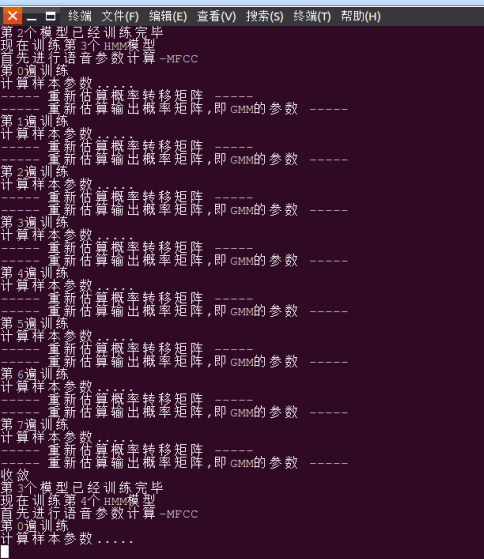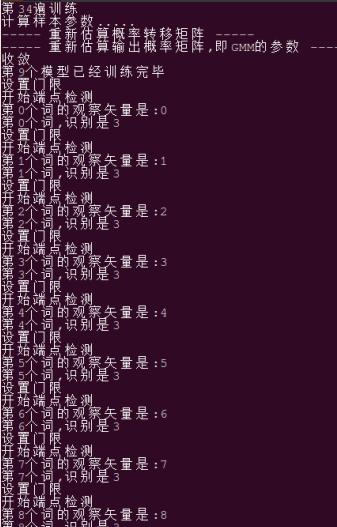1：扫如下支付宝或微信二维码,支付5元

2：把支付单号的后6位，以邮件发送到我的邮箱middleautumn@foxmail.com

3：您也可以在下方留言，把订单号写上来，我会核实。```
```

2014-07-30 21:28:39 Vintage_1 阅读数 5790
• ###### 自然语言处理——实战分词

1245 人正在学习 去看看 魏俊
在跑通Android离线语音识别demo PocketSpinxAndroiDemo后，发现其使用pocketsphinx进行语音识别的准确率并不是很低。这和pocketsphinx语音识别所用的语言模型和声学模型相关。pocketsphinx-0.8源码自带几个语言模型和声学模型，pocketsphinx-0.7/model/hmm下的是声学模型（hmm应该指的是隐马尔科夫模型），pocketsphinx-0.7/model/lm下的是语言模型（lm表示language model）。运行demo时使用的是美国英语的语言模型(/lm/en_US/hub4.5000.DMP)和声学模型(/hmm/en_US/hub4wsj_sc_8k)以及字典文件(/lm/en_US/hub4.5000.dic)，/pocketsphinx/model目录 内容如下，
├── hmm
│   ├── en
│   │  └── tidigits
│   │      ├── feat.params
│   │      ├── mdef
│   │      ├── means
│   │      ├── sendump
│   │      ├── transition_matrices
│   │       └── variances
│   ├── en_US
│   │  └── hub4wsj_sc_8k
│   │      ├── feat.params
│   │      ├── mdef
│   │      ├── means
│   │      ├── noisedict
│   │      ├── sendump
│   │      ├── transition_matrices
│   │      └── variances
│   └── zh
│       └── tdt_sc_8k
│           ├── feat.params
│           ├── mdef
│           ├── means
│           ├── noisedict
│           ├── sendump
│           ├── transition_matrices
│           └── variances
└── lm
├── en
│  ├── tidigits.dic
│  ├── tidigits.DMP
│  ├── tidigits.fsg
│  ├── turtle.dic
│  └── turtle.DMP
├── en_US
│  ├── cmu07a.dic
│  ├── hub4.5000.DMP
│  └── wsj0vp.5000.DMP
├── zh_CN
│  ├── gigatdt.5000.DMP
│  └── mandarin_notone.dic
└── zh_TW
├── gigatdt.5000.DMP
└── mandarin_notone.dic

此外，CMU sphinx的官网提供了各种语言的声学模型和语言模型的下载，具体见，
http://sourceforge.net/projects/cmusphinx/files/Acoustic%20and%20Language%20Models/

Demo用的字典太大，相应的语言模型也很大，而这个语言模型和字典并非针对你而训练的，这是造成识别率低下的主要原因。因此，下面创建自己的语料库drone_ctr.txt，文件的内容是，
take off
land
turn left
turn right
forward
backward
spin left
spin right
up
down
hover

c.setString("-hmm",
"/sdcard/Android/data/edu.cmu.pocketsphinx/hmm/en_US/hub4wsj_sc_8k");
c.setString("-dict",
"/sdcard/Android/data/edu.cmu.pocketsphinx/lm/1172.dic");
c.setString("-lm",
"/sdcard/Android/data/edu.cmu.pocketsphinx/lm/1172.lm");

经过试验，发现准确率在不改变字典的情况下仍然不高，这说明声学模型不变的情况下，字典范围得足够小才能准确识别。

和英文情况类似，先创建语料库drone_ctr.txt，文件的内容是，

c.setString("-hmm",
"/sdcard/Android/data/edu.cmu.pocketsphinx/hmm/zh/tdt_sc_8k");
c.setString("-dict",
"/sdcard/Android/data/edu.cmu.pocketsphinx/lm/9930.dic");
c.setString("-lm",
"/sdcard/Android/data/edu.cmu.pocketsphinx/lm/9930.lm");

http://blog.csdn.net/zouxy09/article/details/7942784语言/声学模型介绍）
http://blog.csdn.net/zouxy09/article/category/1218766Sphinx很好很全面的资料，工具使用、API编程等
http://zuoshu.iteye.com/blog/1463867  （修改Demo的语言/声学模型，英文识别）
http://www.cnblogs.com/yin52133/archive/2012/07/12/2588201.html#2525875  （中文识别解决方案）